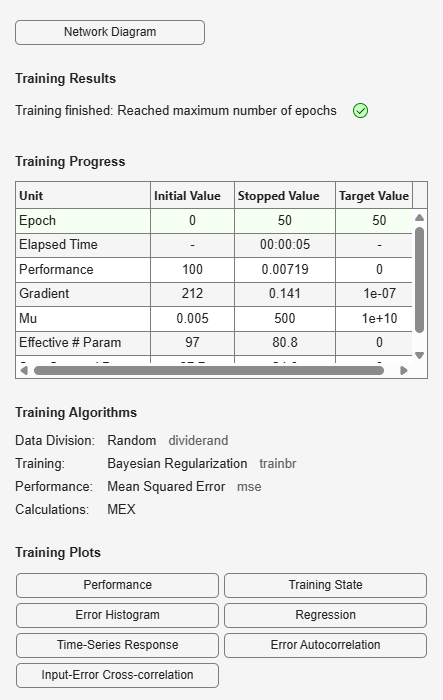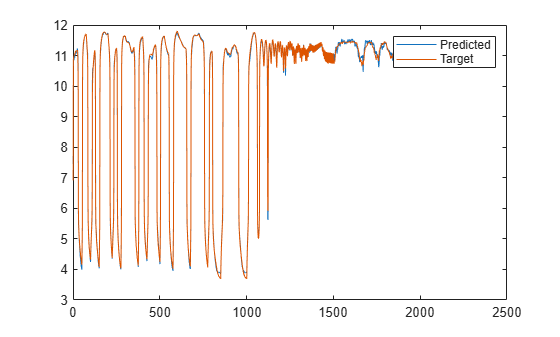## Design Layer-Recurrent Neural Networks

The next dynamic network to be introduced is the Layer-Recurrent Network (LRN). An earlier simplified version of this network was introduced by Elman [Elma90]. In the LRN, there is a feedback loop, with a single delay, around each layer of the network except for the last layer. The original Elman network had only two layers, and used a `tansig` transfer function for the hidden layer and a `purelin` transfer function for the output layer. The original Elman network was trained using an approximation to the backpropagation algorithm. The `layrecnet` command generalizes the Elman network to have an arbitrary number of layers and to have arbitrary transfer functions in each layer. The toolbox trains the LRN using exact versions of the gradient-based algorithms discussed in Multilayer Shallow Neural Networks and Backpropagation Training. The following figure illustrates a two-layer LRN.The LRN configurations are used in many filtering and modeling applications discussed already. To show its operation, this example uses the “phoneme” detection problem discussed in Design Time Series Distributed Delay Neural Networks. Here is the code to load the data and to create and train the network:

```load phoneme p = con2seq(y); t = con2seq(t); lrn_net = layrecnet(1,8); lrn_net.trainFcn = 'trainbr'; lrn_net.trainParam.show = 5; lrn_net.trainParam.epochs = 50; lrn_net = train(lrn_net,p,t);```After training, you can plot the response using the following code:

```y = lrn_net(p); plot(cell2mat(y))```The plot shows that the network was able to detect the “phonemes.” The response is very similar to the one obtained using the TDNN.

Each time a neural network is trained, can result in a different solution due to different initial weight and bias values and different divisions of data into training, validation, and test sets. As a result, different neural networks trained on the same problem can give different outputs for the same input. To ensure that a neural network of good accuracy has been found, retrain several times.

There are several other techniques for improving upon initial solutions if higher accuracy is desired. For more information, see Improve Shallow Neural Network Generalization and Avoid Overfitting.